# Xbar and s (Xbar-s) Chart

## Chart Components

As in the Xbar-R chart, the Xbar chart (the upper chart in this figure) plots the average of individual values in a subgroup (i.e., the subgroup mean). The s chart (the lower chart in the figure) plots the sample standard deviation of the individual values in the subgroup.
This chart is sometimes referred to as Xbar-SD.

### Xbar-s Charts for a Single Characteristic

Traditionally, an Xbar-s chart is used to plot the subgroup mean for a larger subgroup and the standard deviation.

For example, this sample chart (taken from InfinityQS® ProFicient™ software) highlights subgroup 9 of 20 subgroups. You can see that the average of the subgroup’s plot points is 34.02 (top chart) and the standard deviation is 2.755 (lower chart).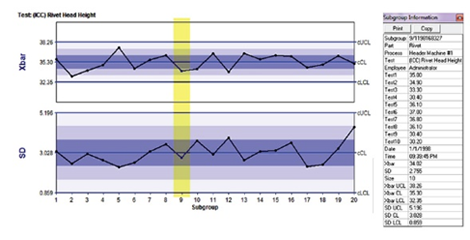## How to Use the Xbar-sChart

Use the Xbar-s chart chart when your sample size is 10 or more (n≥10). This scenario is most common when a lot of data is available and the cost for gathering it is low.

For example, you might use this chart for data taken from Programmable Logic Controllers (PLCs) or other automated data-collection devices. Injection molding, multihead fill operations, and continuous high-speed production lines on which many measurements can be gathered quickly and affordably are all good environments for this type of chart.

Each of the special uses described on this page presume a large sample size (i.e., 10 or more).

• Very sensitive to small changes in the subgroup mean
• Standard deviation is usually a more accurate indicator of process variation than is the range
• Requires gathering large amounts of data to calculate control limits

## Decision Tree

Use the following decision tree to determine whether the Xbar-s chart is the best choice.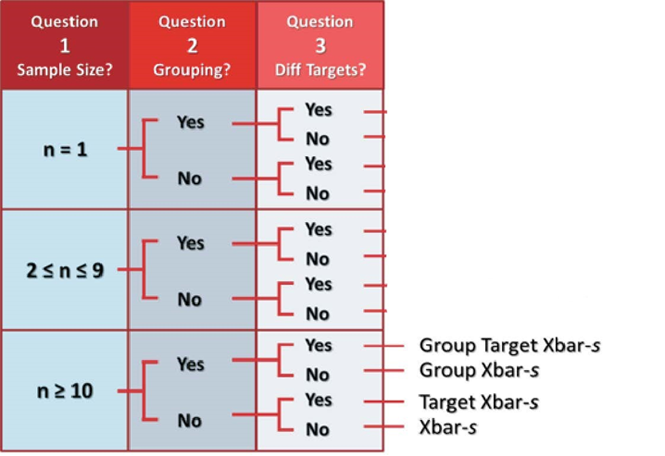## Special Uses

Today, control charts are a key tool for quality control and figure prominently in Lean manufacturing and Six Sigma efforts.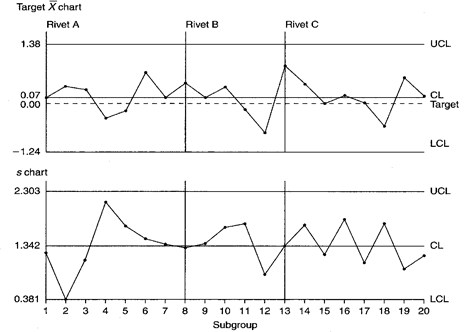### Target Xbar-s Chart

Target Xbar-s charts can help you identify changes in the average and standard deviation of a characteristic. You can measure the characteristic across part numbers, but each part number must form a separate subgroup because target values change with the part number. Set the target values at the desired center, typically the center two-sided specifications.
• Plot multiple parts or characteristics with similar variability on the same chart.
• Assess statistical control for the process as well as for each of its parts or characteristics.
• Detect very small process shifts.
• Directly plot data from gauges that are zeroed out on target values (no data transformation or coding necessary).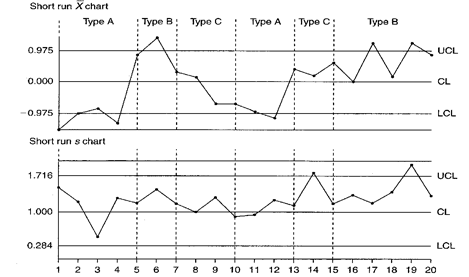### Short Run Xbar-s Chart

Short run charts are used for short production runs. The short run Xbar-s chart can help you identify changes in the averages and standard deviation of multiple characteristics, even those with different nominals, units of measure, or standard deviations.

• Summarize a great amount of data while still detecting small changes in process average.
• Detect the difference between process- and product-specific variabilities.
• Plot variations of multiple products, even those with differing standard deviations, nominals, or units of measure—all on one chart.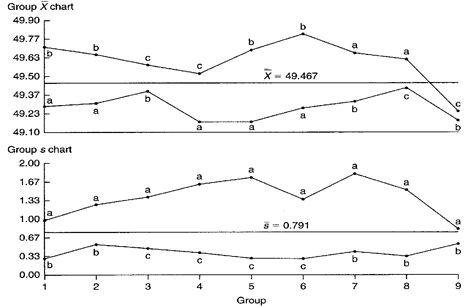### Group Xbar-s Chart

Group charts display several parameters, characteristics, or process streams on one chart. Group Xbar-s charts help you assess changes in averages and the standard deviation across measurement subgroups for a characteristic.

• Compare the variations of a variety of products or characteristics.
• See the difference between variations that are caused by changes in average and those caused by changes in the standard deviation.
• Clearly detect characteristics that are priorities for attention.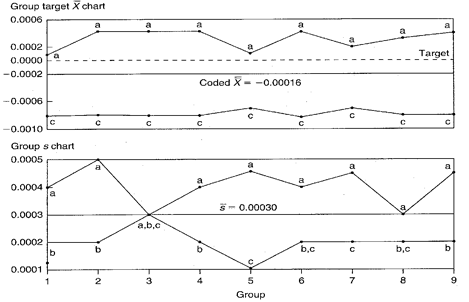### Group Target Xbar-s Chart

The group target Xbar-s chart provides information about changes in process averages and the standard deviation across multiple measurement subgroups of similar characteristics that have a common process. Part numbers and engineering nominal values can differ across these characteristics.

• Compare variations of multiple products or characteristics as well as similar characteristics with different averages.
• See the difference between variations that are caused by changes in average and those caused by changes in the standard deviation.### Group Short Run Xbar-s Chart

The group short run Xbar-s chart enables you to spot changes in the process average and standard deviation across multiple characteristics in a short run environment.
• Identify the difference between process- and product-specific variations.
• Compare variations of multiple products or characteristics.
• Analyze characteristics from a variety of parts, even those with different means, standard deviations, or units of measure.
• See the difference between variations that are caused by changes in average and those caused by changes in the standard deviation.

### Take the first step from quality to excellence

Take the Next Steps